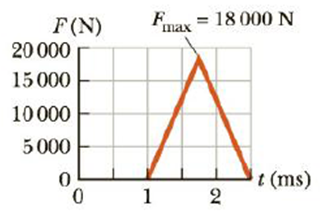Chapter 6, Problem 8P

Chapter
Section
Textbook Problem

An estimated force vs. time curve for a baseball struck by a bat is shown in Figure P6.8. From this curve, determine (a) the impulse delivered to the ball and (b) the average force exerted on the ball.(a)

To determine
The impulse delivered to the ball.

Explanation

The below graph represents the force versus time curve for a baseball struck by the bat,

Formula to calculate the time taken by the ball from the graph is,

Δt=X2X1

• X2 is the final time.
• X1 is the initial time.
• Δt is the time taken by the ball.

Use the plotted points from the graph,

Substitute 2.50s for X2 and 1.0s for X1 in the above equation to find Δt ,

Δt=2.50ms1.0ms=1.50ms

The impulse delivered to the ball is given in the force and time ratio by graph and it is equal to the area under the curve formed by the force and time in the graph. The area in the curve is as triangle.

Formula to calculate the area of triangle is,

A=12Δta

• A is the area of the triangle,
• a is the altitude.

Formula to calculate the impulse delivered to the ball,

Consider area of the triangle is equal to the impulse delivered to the ball

(b)

To determine
The average force exerted on the ball.

Still sussing out bartleby?

Check out a sample textbook solution.

See a sample solution

The Solution to Your Study Problems

Bartleby provides explanations to thousands of textbook problems written by our experts, many with advanced degrees!

Get Started